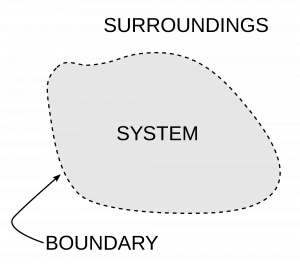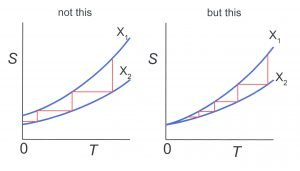Join Our WhatsApp Group!

# The Laws of Thermodynamics – Limitation and Best Concepts

Let’s start the explanation of the Laws of Thermodynamics

## The Laws of thermodynamics

Thermodynamic is principally based on a set of four laws that are universally valid when applied to the system that falls within the constraints implied by each.Explain all Four laws of thermodynamics

### Zeroth law of thermodynamics

The zeroth law of thermodynamics states that if two systems are each in thermal equilibrium with a third, they are also in thermal equilibrium with one another. It implies that thermal equilibrium is an equivalence relation in the set of the thermodynamic systems under consideration. The system is said to be in equilibrium if the small, random exchanges between them (Ex: Brownian motion) do not lead to a net change in energy. The first law provides an empirical definition of temperature and justification for the construction of practical thermometers.

Internal Energy: It is the sum of molecular kinetic and potential energies in the frame of reference relative to which the center of mass of the system is at rest.

• The intermolecular potential energy of a real gas is a function of its volume.
• The internal energy of a gas is a function of its temperature.
• The internal energy of a system is a thermodynamic state variable.
• The internal energy of an ideal gas is purely kinetic in nature.
 Heat Work 1.   Heat is a mode of energy transfer that produces random motion. 2.   Heat is a mode of energy transfer due to temperature differences in the system and the surroundings. 1.   Work is the mode of energy transfer bought about by means that do not involve temperature differences. 2.   Work may be regarded as the mode of energy transfer that produces organized motion.

The sign convention used:

• Heat observed by a system is positive. Heat given out by a system is negative (-ve).
• Work done by a system is positive. Work done on a system is negative (-ve).
• The increase in the internal energy of a system is positive. The decrease in the internal energy of a system is negative.

### The first law of thermodynamics

1st law of thermodynamic states that the internal energy of an isolated system is constant. The first law is an expression of the principle of the conservation of energy which is usually formulated by assuming that the change in the internal energy of a closed thermodynamic system is equal to the difference between the heat supplied to the system and the amount of work done by the system on its surroundings. It is important to note that internal energy is a state of the system. In other words, the specific internal energy of the system may be achieved by any combination of heat and work; the manner by which a system achieves specific internal energy is path-independent.#### Limitation of the first law of Thermodynamics

• Thermodynamic first law does not tell anything about the condition under which heat can be converted into mechanical work.
• Thermodynamic 1st law does not indicate the extent to which heat energy can be converted into mechanical work continuously.
• The first law of thermodynamic does not indicate the direction of the transfer of heat.

### The second law of thermodynamics

2nd law of thermodynamics states that heat cannot spontaneously flow from a colder location to a hotter location. It being more an expression of the universal principle of decay observable in nature explains the phenomenon of irreversibility in nature. There are many processes in which energy is converted and yet they are never observed. The principle which disallows such phenomena consisted of the first law of thermodynamics is called the second law of thermodynamics. It can be stated in a number of ways:

Kelvin-Planck statement: It is impossible to construct an engine, which will produce no effect other than extracting heat from a reservoir and performing an equivalent amount of work.

Clausius statement: It is impossible for self-acting machines, unaided by any external agency, to transfer heat from a body to another at a higher temperature.

#### Significance of second law

• According to the second law, the coefficient of performance of a refrigerator can never be infinite.
• According to the second law, the coefficient of a heat engine can never be unity.

### Third law of Thermodynamics

3rd law of thermodynamic states that as a system approaches absolute zero, all processes cease, and the entropy of the system approaches a minimum value. The third law of thermodynamics provides an absolute reference point for the determination of entropy. The entropy determined relative to this point is the absolute entropy. The entropy of all systems and of all states of a system is the smallest at absolute zero, or equivalently however it is impossible to reach the absolute zero of temperature by any finite number of processes. Absolute zero refers to the temperature at which all activity would stop if it were possible to happen. It is -273.16 degrees Celsius or -459.67 degrees Fahrenheit or 0 K.I hope you like this post “The Laws of Thermodynamics”

Like this post and comment below for other Topic

View more…

### 2 thoughts on “The Laws of Thermodynamics – Limitation and Best Concepts”

1.This content is very simple and useful
2.